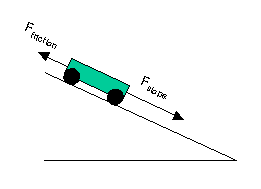# Solutions to SQA examination

### Paper I Solutions

```1. E		11. E			21. D
2. C		12. C			22. D
3. B		13. D			23. A
4. D		14. D			24. E
5. A		15. B			25. D
6. B		16. C			26. E
7. B		17. D			27. E
8. D		18. D			28. A
9. E		19. E			29. A
10.A		20. C			30. C

31.	u = 0m/s
s = 400
t = 17.5s
a = ?

s = ut + 1/2(at2)
400 = 0 + 0.5xa17.52
a = 400/153.25
a = 2.6m/s2

32.	Fslope = mgsinq
Fslope = 2.0x9.8sin30o
Fslope = 9.8NFresultant = Fslope - Ffriction
Fresultant = 9.8-4.0
Fresultant = 5.8N

33.	P1 = 1.6x107Pa
V1 = 0.060m3
P2 = 2.5x105Pa
V2 = ?

P1V1 = P2V2
V2 = P1V1/P2
V2 = 3.84m3

34.	E = QV
E = 5x12
E = 60J

35.	The ratio Vc/Vs will decrease.

The current in the circuit increases as the frequency of the supply is
increased.  This means the potential difference across the resistor(VR)
must have increased. Furthermore, as Vc decreases as VR increases,
the ratio Vc/Vs must decrease, if Vs is held constant.

36.a.	The student requires to know :

i.   the initial temperature of the lead;
ii.  the mass of ice melted as a result of placing the lead
block in the ice; and
iii. the specific latent heat of fusion of water.

NB. Assume the lead block cools down to a temperature of 0oC.

b.	Some of the melted ice is a result of heat from the surroundings
and not from the lead. Not allowing for this, by setting up a control
experiment, will increase the calculated amount of energy released by
the lead. This will in turn lead to a specific heat capacity greater than
the accepted value.

37.a.	dsinq = nl

d = (1/300)mm = 0.00333mm = 3.333x10-6m
n = 2
q = 23o
l = ?

l = dsinq/n
l = 3.333x10-6sin23o/2
l = 651nm

b. 	Red

38.	Mean t1/2 = Total/N

Mean t1/2 = (53.0+54.1+57.5+56.3+55.1)/5
Mean t1/2 = 276/5
Mean t1/2 = 55.2s

Random error = (max - min)/N
Random error = (57.5 - 53.0)/5
Random error = 4.5/5
Random error = 0.9s

t1/2 = (55.2+-0.9)s

END OF QUESTION PAPER